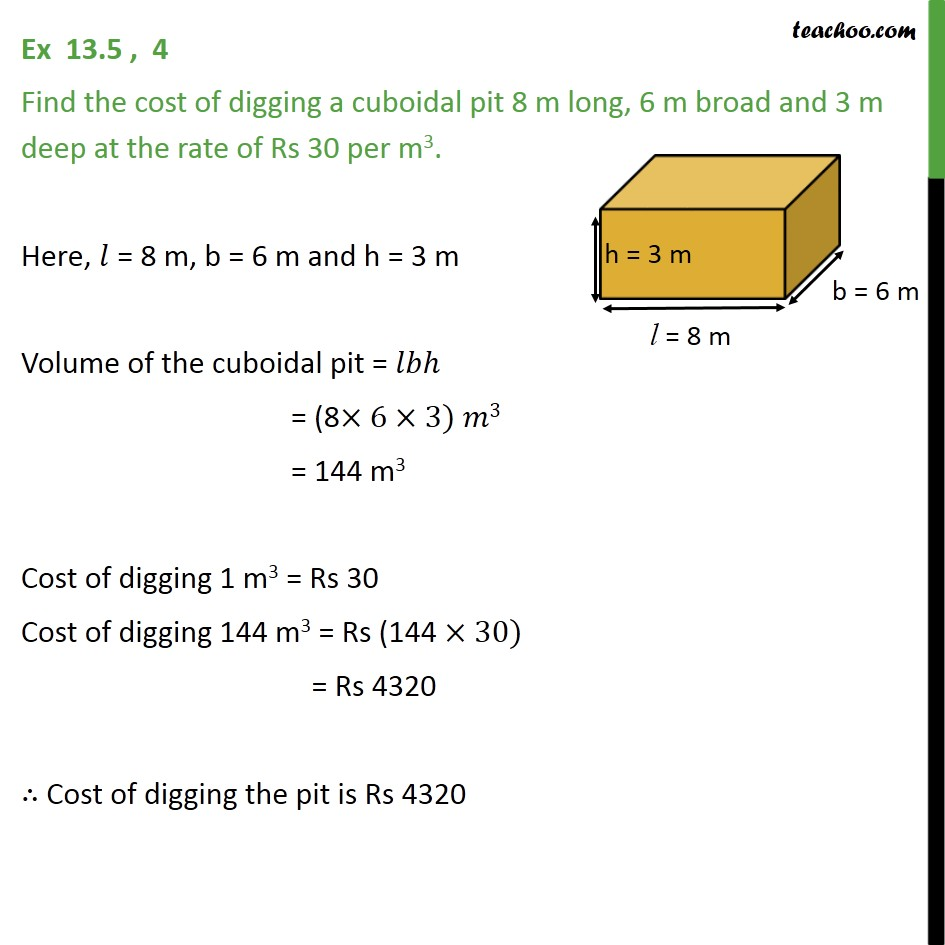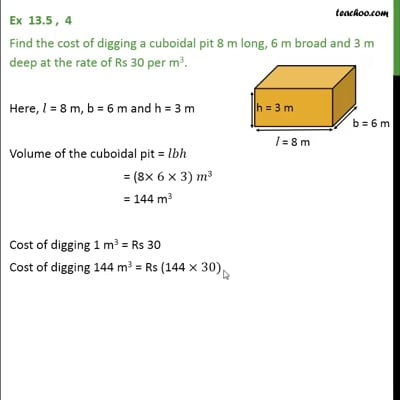Ex 13.5

Chapter 13 Class 9 Surface Areas and Volumes
Serial order wiseThis video is only available for Teachoo black users

Maths Crash Course - Live lectures + all videos + Real time Doubt solving!

### Transcript

Ex 13.5 , 4 Find the cost of digging a cuboidal pit 8 m long, 6 m broad and 3 m deep at the rate of Rs 30 per m3. Here, 𝑙 = 8 m, b = 6 m and h = 3 m Volume of the cuboidal pit = 𝑙𝑏ℎ = (8×6×3) 𝑚3 = 144 m3 Cost of digging 1 m3 = Rs 30 Cost of digging 144 m3 = Rs (144 ×30) = Rs 4320 ∴ Cost of digging the pit is Rs 4320